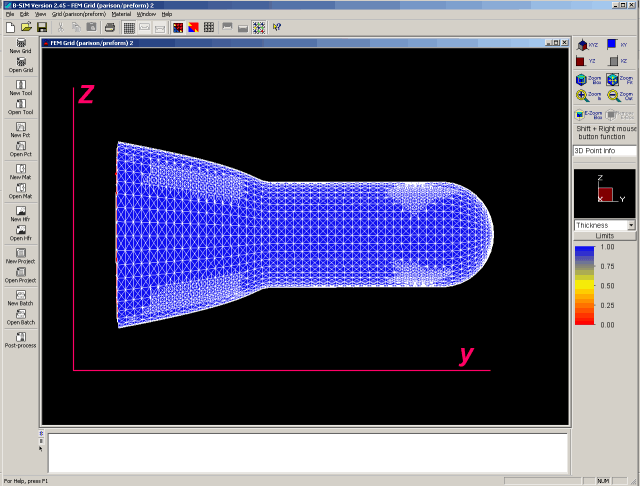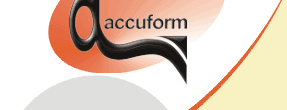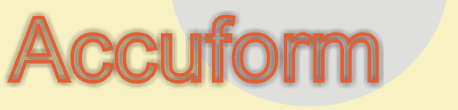B-SIM V2.5 Reference - Blow molding simulationImporting 3D preform - Requirements and details The routine for import of general 3D preforms supports the following file formats: STL, DXF, TOL (Accuform native tool format), VRML, PATRAN, HYPERMESH ASCII. There are two possibilities how to import the preform: 1) Using one file, containing the outer and inner surface, represented by triangles located in 3D space. The triangles must be connected by their edges. B-SIM analyzes the triangles to find out two base curves. The base curves are created from those triangle edges whose do not have any neighbor triangle (i.e. from "outer" edges). If this procedure fails, 3D preform cannot be converted to shell elements grid and B-SIM displays an error message. The base curves are searched in z = z min plane, so make sure your preform geometry is oriented properly. If you are not sure about the orientation or you simple cannot modify your existing file, import this file into B-SIM as tool, do the necessary transformations, and save the file as B-SIM tool. Then repeat the import procedure, now use the new TOL file as an input. 2) Using two files, containing the outer and inner surface separately. The surfaces are represented by triangles located in 3D space. The triangles must be connected by their edges. B-SIM analyzes the triangles to find out two base curves. The base curves are created from those triangle edges whose do not have any neighbor triangle (i.e. from "outer" edges). If this procedure fails, 3D preform cannot be converted to shell elements grid and B-SIM displays an error message. The base curves are searched in z = z min plane, so make sure your preform geometry is oriented properly. If you are not sure about the orientation or you simple cannot modify your existing files, import the files into B-SIM as tools, do the necessary transformations, and save the files as B-SIM tools. Then repeat the import procedure, now use the new TOL files as an input. Why the import fails... The following message is displayed if the import routine fails: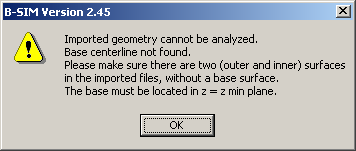The base curves problem Typically, if the grids are OK (no holes, triangles connected), the base curves do not fulfill the requirement to lay in z = z min plane. The solution is to make the necessary geometrical transformations in order to have the base curves in z = z min. Use your CAD to transform the surfaces (triangles) or import the file(s) into B-SIM as tool(s), perform the transformations and save the tools (as TOL files). Then use the file(s) again. Example:This picture shows an imported 3D grid. The outer surface is blue, the inner is red. Surfaces were imported from separate files. However, during base curves search, it was found that the curves do not lay in z = z min plane. As it is obvious, the blue (outer) surface is higher tan the red (inner) surface. The solution is to move the outer surface in the direction of negative z axis to have both base curves at z = z min. Closed base problem B-SIM requires the base to be open. There cannot be any elements laying in z = z min plane, as these elements create a connection between outer and inner surface and the importing routine fails when making decision what surface is inner and what outer. In general, the outer and inner surfaces cannot be found. As a result, the base curves cannot be found, too.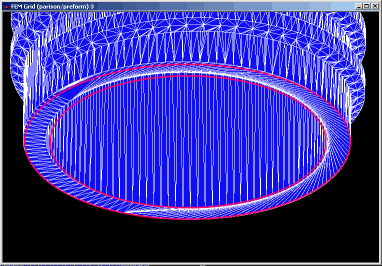In the image above, the imported preform contains the base surface (between pink curves). The base elements must be removed. Use your CAD or import the preform as tool into B-SIM and use Tool / Cut tool command to remove the redundant triangles. Save the tool as TOL and use the new file for import again. Wrong orientation problem B-SIM requires the base to be at z = z min. This means the preform must be oriented along z axis, having its opening at z = z min. You can use your CAD to transform the preform, or import the preform as tool into B-SIM, make the transformations, save the file as TOL and use the new file for import. The following image shows wrong orientation problem: